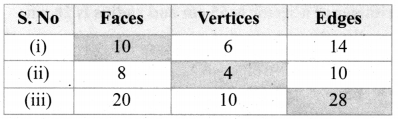Tamilnadu State Board New Syllabus Samacheer Kalvi 8th Maths Guide Pdf Chapter 2 Measurements Ex 2.4 Text Book Back Questions and Answers, Notes.

## Tamilnadu Samacheer Kalvi 8th Maths Solutions Chapter 2 Measurements Ex 2.4

Question 1.
Two gates are fitted at the entrance of a library. To open the gates easily, a wheel is fixed at 6 feet distance from the wall to which the gate is fixed. If one of the gates is opened to 90°, find the distance moved by the wheel (π = 3.14).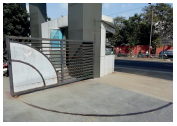Amswer:
Let A be the position of the wall AC be the gate in initial position and AB be position when it is moved 90°.
Now the arc length BC gives the distance moved by the wheel.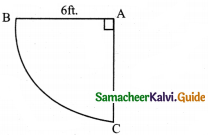Length of the arc = $$\frac{\theta}{360^{\circ}}$$ × 2πr units
= $$\frac{90^{\circ}}{360^{\circ}}$$ × 2 × 3.14 × 6 feets
= 3.14 × 3 feets
= 9.42 feets
∴ Distance moved by the wheel = 9.42 feets.Question 2.
With his usual speed, if a person covers a circular track of radius 150 m in 9 minutes, find the distance that he covers in 3 minutes (π = 3.14).
Radius of the circular track = 150m
Distance covers in 9 minutes = Perimeter of the circle = 2 × π × r units
Distance covered in 9 min = 2 × 3.14 × 150m
Distance covered in 1 min = $$\frac{2 \times 3.14 \times 150}{9} \mathrm{m}$$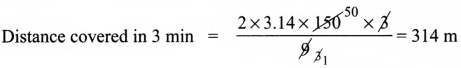Distance he covers in 3min = 314m

Question 3.
Find the area of the house drawing given in the figure.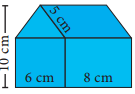Area of the house = Area of a square of side 6 cm + Area of a rectangle with l = 8cm, b = 6 cm + Area of a ∆ with b = 6 cm and h = 4 cm + Area of a parallelogram with b = 8 cm, h = 4 cm
= (side × side) + (l × b) + ($$\frac { 1 }{ 2 }$$ × b × h) + bh cm2
= (6 × 6) + (8 × 6) + ($$\frac { 1 }{ 2 }$$ × 6 × 4) + (8 × 4) cm2
= 36 + 48 + 12 + 32 cm2
= 128 cm2
Required Area = 128 cm2Question 4.
Draw the top, front and side view of the following solid shapes
(i)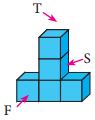(a) Top view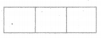(b) Front view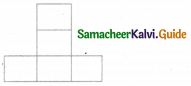(c) Side view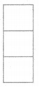(ii)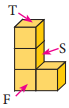(a) Top view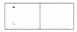(b) Front view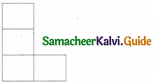(c) Side view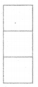Challenging ProblemsQuestion 5.
Guna has fixed a single door of width 3 feet in his room where as Nathan has fixed a double door, each of width 1 $$\frac { 1 }{ 2 }$$ feet in his room. From the closed position, if each of the single and double doors can open up to 120°, whose door takes a minimum area?
Width of the door that Guna fixed = 3 feet.
When the door is open the radius of the sector = 3 feet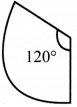Angle covered = 120°
∴ Area required to open the door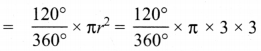= 3π feet2

(b) Width of the double doors that Nathan fixed = 1 $$\frac { 1 }{ 2 }$$ feet.
Angle described to open = 120°
Area required to open = 2 × Area of the sector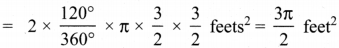z
= $$\frac { 1 }{ 2 }$$ (3π) feet2
∴ The double door requires the minimum area.Question 6.
In a rectangular field which measures 15 m x 8m, cows are tied with a rope of length 3m at four corners of the field and also at the centre. Find the area of the field where none of the cow can graze. (π = 3.14).
Area of the field where none of the cow can graze = Area of the rectangle – [Area of 4 quadrant circles] – Area of a circle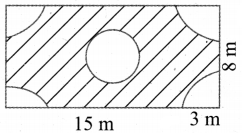Area of the rectangle = l × b units2
= 15 × 8m2 = 120m2
Area of 4 quadrant circles = 4 × $$\frac { 1 }{ 4 }$$ πr2 units
Area of 4 quadrant circles = 4 × $$\frac { 1 }{ 4 }$$ × 3.14 × 3 × 3 = 28.26 m2
Area of the circle at the middle = πr2 units
= 3.14 × 3 × 3m2 = 28.26m2
∴ Area where none of the cows can graze
= [120 – 28.26 – 28.26]m2 = 120 – 56.52 m2 = 63.48 m2Question 7.
Three identical coins, each of diameter 6 cm are placed as shown. Find the area of the shaded region between the coins. (π = 3.14) (√3 = 1.732)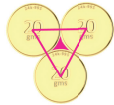Given diameter of the coins = 6 cm
∴ Radius of the coins = $$\frac { 6 }{ 2 }$$ = 3 cm
Area of the shaded region = Area of equilateral triangle – Area of 3 sectors of angle 60°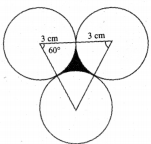Area of the equilateral triangle = $$\frac{\sqrt{3}}{4}$$ a2 units2 = $$\frac{\sqrt{3}}{4}$$ × 6 × 6 cm2
= $$\frac{1.732}{4}$$ × 6 × 6 cm2 = 15.588 cm2
Area of 3 sectors = 3 × $$\frac{\theta}{360^{\circ}}$$ × πr2 sq.units
= 3 × $$\frac{60^{\circ}}{360^{\circ}}$$ × 3.14 × 3 × 3 cm2 = 1.458 cm2
∴ Area of the shaded region = 15.588 – 14.13 cm2 = 1.458 cm2
Required area = 1.458 cm2 (approximately)Question 8.
Using Euler’s formula, find the unknowns.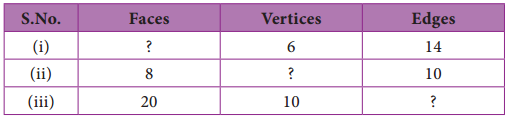Euler’s formula is given by F + V – E = 2
(i) V = 6, E = 14
By Euler’s formula = F + 6 – 14 = 2
F = 2 + 14 – 6
F = 10

(ii) F = 8,E = 10
By Euler’s formula = 8 + V – 10 = 2
V = 2 – 8 + 10
V = 4(iii) F = 20, V = 10
By Euler’s formula = 20 + 10 — E = 2
30 – E = 2
E = 30 – 2.
E = 28
Tabulating the required unknowns